## Mathematics Paper 1 Questions and Answers - Arise and Shine Trial Mock Exams 2022

Instructions to Candidates

• Write your name and index number in the space provided above.
• Sign and write the date of examination in the space provided above.
• This paper consists of TWO sections: section I and section II
• Answer all the questions in section I and on only five questions from section II
• Show all the steps in your calculations, giving your answers at each stage in the spaces provided below each question.
• Marks may be given for correct marking even if the answer is wrong
• Non-programmable silent calculator and KNEC Mathematical tables may be used, except where stated otherwise.
• Candidates should check the question paper to ascertain that all the pages are printed as indicated and that no questions are missing.
• Candidates should answer the questions in English

For Examiner’s Use Only
Section I

 1 2 3 4 5 6 7 8 9 10 11 12 13 14 15 16 Total

Section II

 17 18 19 20 21 22 23 24

### QUESTIONS

SECTION I [50 marks]
Answer all the questions in this section in the spaces provided.

1. Without using a calculator, evaluate. (3 marks)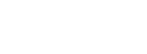2. Three bells ring at intervals of 9 minutes, 15 minutes and 21 minutes. The bells will net ring together at 11.00p.m. Find the time the bells had last rang together. (3 marks)
3. Simplify: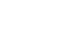(2 marks)
4. Solve 4 ≤3x- 2 < 9 + x; hence list the integral values that satisfies the inequality. (3 marks)
5. The sum of interior angles of two regular polygons of side (n-1) and n are in the ratio 4:5. Calculate the value of interior angle of the polygon with side (n-1). (3 marks)
6. A bus travelling at a speed of 63km/h left a station at 8.15am. a car later left the same station at 9.00 am and caught up with the bust at 10.45am. Find the average speed of the car. (3 marks)
7. Given that r=2 and h = 3r-1,evaluate (3 marks)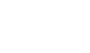8. A line L Passes through point (3, 1) is perpendicular to the line 2y = 4x + 5. Determine the equation of Line L. (3 marks)
9. The figure shows part of triangular Prism. ABCDEF. Complete the prism and measure length AF. (4mark)10. The position vectors of A,B and C are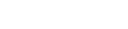respectively. Vector CA is parallel to vector OB. Determine the value of a. (3 marks)
11. A Kenyan company received US dollars 100,000. The money was converted into Kenya shillings in a bank which buys and Sells foreign currencies as follows.
 Buying  (Ksh) Selling(Ksh) I  US dollar 77.24 77.44 I Sterling Pound 121.93 122.27
1. Calculate the amount of money, in Kenya shillings, the company received. (2 marks)
2. The company buys a car from Britain in Sterling Pound. Calculate the cost of the car to the nearest Sterling Pound. (2 marks)
12. Convert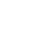into degrees. (2 marks)
13. Onyango travelled by train from Kisumu to Nairobi. The train left Kisumu on a Sunday at 2350 hour and travelled for 7 hours 15 minutes to reach Nakuru. After a 45 minutes stop in Nakuru, the train took 5 hours 40 minutes to reach Nairobi. Find the time in the 12 hours clock system and the day Onyango arrived in Nairobi. (3 marks)
14. Two containers have base areas of 750cm2 and 120cm2 respectively. Calculate the volume of the larger container in litres given that the volume of the smaller container is 400cm3. (3 marks)
15. The figure below represents a plot of land ABCD such that AB=85m, BC = 75m, CD = 60m, DA = 50m and angle ACB = 90º. (3 marks)Determine the area of the plot in hectares correct to two decimal places. (4 marks)
16. Use reciprocal to find the reciprocal of 0.01732. Hence evaluate. (3 marks)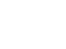SECTION II – (50 Marks)
Answer Only Five Questions in this section in the spaces provided.

1. A newly built classroom measuring 6.3m long, 4.5 wide and 3.2m high is to be cemented on the floor and all inside walls. The classroom has one door measuring 1.85m by 80cm and four windows measuring 1.5m by 70cm each. Cementing materials cost sh 500 per square metre while labour costs 20% of the cost of cementing materials. Calculate:-
1. to one decimal place, the total surface area to be cemented. (4 marks)
2. The cost of cementing materials. (2 marks)
3. The total cost of cementing the classroom. (2 marks)
4. The school borrowed the loan to facilitate the total cost of cementing the classroom from a bank. The money earned a compound interest of 8% per annum. How much did the school repaid after 3 years. (2 marks)
2. In the figure below (not drawn to scale) AB = 8cm, AC = 6cm, AD = 7cm, CD = 2.82cm and ∠CAB = 50º.Calculate, to 2 decimal places:-
1. The length BC (2 marks)
2. The size of ∠ABC; (3 marks)
3. The size of ∠CAD (3 marks)
4. the area of triangle ACD. (2 marks)
3.
1. Two matrices P and Q are such that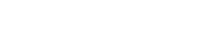. Given that the determinant of PQ = 4, find the value of k. (3 marks)
2. Find M-1 the inverse of matrix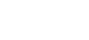(2 marks)
3. Omondi bought 5 plates and 6 mugs for a total of ksh 2440. Ali bought 7 plates and 9 mugs for a total of Ksh 3560.
1. Form a matrix equation to represent the above information. (1 mark)
2. Use matrix method to find out the cost of a plate and that of a mug. (4 marks)
4.
1. Solve the equation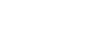(4 marks)
2. The length of a floor of a rectangular hall is 9m more than its width. The area of the floor is 36m2.
1. Calculate the perimeter of the floor. (4 marks)
2. A rectangular carpet is placed on the floor of the hall leaving an area of 28m2. If the length of the carpet is twice its width, determine the width of the carpet. (2 marks)
5. Three vertices of a parallelogram ABCD are A (-7,3), B(1,-1) and C(5,1). On the grid provided, draw the parallelogram ABCD. (2 marks)Determine
1. the gradient of the line AB. (2 marks)
2. the equation of line AB in the form y = mr + c, where m and c are constants. (2 marks)
3. Another line L is perpendicular to CD and passes through point (1, 3),
Determine:
1. the equation of L in the form ax+ by = c where a, b and c are constants. (3 marks)
2. the coordinates of the y-intercept of line L. (1 mark)
6. The marks of 40 students in a mathematics examinations were recorded as follows
64 50 58 73 51 42 58 46
58 60 45 48 69 48 50 43
52 64 58 46 59 54 41 61
73 49 74 55 44 73 53 67
62 47 66 52 60 61 54 70
1. Complete the frequency distribution table be low for the above information. Use classes of size 5 starting with the class 40-44. (4 marks)
 Mass (kg) Frequency(f) Mid-points(x) fx cf 40-44
2. State the modal class. (1 mark)
3. Estimate:
1. the mean mark. (2 marks)
2. the median mark. (3 marks)
7. The vertices of trapezium ABCD are A (2, 0), B (4, 0), C (6, 2), and D (2, 2)
1. On the grid provided below, draw:
1. the trapezium ABCD; (1 mark)2. A’B’C’D’ the image of ABCD under a reflection in the line y = -x; (2 marks)
3. A”B”C”D” the image of A’B’C’D’ under rotation of -90o, centre (0, 0). (2 marks)
2. Describe a transformation that maps A”B”C”D” onto ABCD (2 marks)
3. State pairs of trapezia that are directly congruent and those that are oppositely congruent. (3 marks)
8. The gradient of the curve y = 2x3 – 9x2 + px + 1
1. Find
1. the value of P (3 marks)
2. the equation of the tangent to the curve at x = 0.5. (4 marks)
3. Find the co-ordinates of the turning points of the curve. (3 marks)

### MARKING SCHEME

1

-2 (3) – 3-5
= -14
15 – 8= 7
-14/7 = 2

2

LCM 3   9   15   21
3   3    5     7
5   1    5     7
7   1    1     7
32 x 5 x 7 = 315
315 min
5 hrs 15 mins
11.00 p.m
5hrs  15mins
5.45 p.m
NB (deny 5:45 p.m)

3

272/3 ÷ 24
(25) -3/5
(33)2/3 ÷ 24
(25)-3/5
= 32 ÷24
2 - 3
= 32 ÷ 2
= 4.5

4

4 ≤ 3x - 2
6 ≤ 3x
2 ≤ x     B1
3x – 2 < 9 + x
2x < 11
x < 5.5
x: 2,3,4,5,   B1

5

180 (n-1-2) = 4/5
180(n-2)
4n – 8 =5n – 15
n = 7
Interior angle
(6-2)180
6
=120o

6

Distance covered by bus in 45 mins
63 x 45/60  = 47.25km
Let car’s sped 60 xkm/h
R.speed = (x – 63)km/h
Time taken to catch up
1hr 45 mins
47.25 = 1 ¾ (x – 63)
x– 63 = 4/7 (47.25)
=27
x = 100km/h
Accept Aft. method

7

r = 2      h = 3(2)-1 = 58

2y = 4x + 5 ⇒ y = 2x + 2.5
m2
Grad. Of h line = -1/2
= y - 1  = ½
x - 3
-x + 3 = 2y – 2
2y = -x + 5

9B1 shape
B1 – hidden line  dotted
AE/ED and EP
B1 – accurate
Led equal
Ac= 3cm,AB=4cm
Bf=5.5cm

(ii). AF = 9.0cm ± 0.1

10

OB//CA
KOB = CA11

(a).1 US pond = 77.24/=
100,000 x 77.24
= ksh. 7,724,000

(b). 1 US pond = 122.27/=
? 7724,000
7724000
122,27
63,172 starling  pond (nearest)

12

2nc x 360
9     2nc
= 40o

13

(2350 + 07h 15 min + 45 min) + 5 hr 40 min
= 1.330he-
1200
1.30 p.m Monday

1415AC = √852 - 752
= 40m
Area ABC = ½ x 40 x 75 M1
=1500m2
S = 60 + 40 + 50 = 75m
2
Area ADC 75√ (75 - 50) (75 - 60) (75 - 40)
= 992.16m2
= 1500 + 992.16 = 0.25ha A1 (2d.p)
10000
2350+7h 15 min
M1M1 3105 – 2400
= 005+
45 min
0750 hr
0750hr +
0540
1330 hrs
-1200
1.30 p.m, Monday A1

16

Rec of 0.01732
1.732-1 x 102
= 0.5774 x 102
= 57.74
3√0.008
0.01732
3√0.008 x 57.74
= 0.2 x 57.74
= 11.548 A1

SECTION II

17

(a). Area of front & back walls
= 6.3 x 3.2 x 2
= 40.32m2
Area of side walls = 4.5 x 3.2 x 2
= 28.8m2
Area of floor = 6.3 x 4.5
= 28.35m2
Total area = 40.32 + 28.8 + 28.35
= 97.47m2
Area of door          = 1.85 x 0.8 = 1.48m2
Are of windows = 1.5 x 0.7 x 4 = 4.2m2 +
Area of windows cemented       = 5.68m2
Total area  not cemented           = 97.47 – 5.68
= 91.79
= 91.8m2
(b). Cost of cementing materials
= 91.8 x 500
= sh 45,900
c). Cost of labour = 20/100  x 45,900
= sh. 9180

Total cost of cementing
= 45900 + 9180
= sh 55,080

(d). A = P (1 + r/100)n
= 55,080 (1 + 8/100)3
= 55,080 (1.08)3
= Ksh.69,384.93696
= Ksh 69,385

18

(a). BC2 = 62 + 82 – 2 x 6 x 8 cos 50o
BC2 = 100 – 96 cos 50o
BC2 = 100 – 61.71
= √38.29
= 6.19cm

(b).  =   6   =   6.19
sin B   sin 50º
Sin B =  6 sin 50o
6.19

Sin B = 0.7425
B = sin-1 0.7425
= 47.95o

2.822 = 72 + 62 – 2(6x7)cosA

7.9524 = 85 – 84 cos A
Cos A = 85 – 7.9524M1
84
= 0.9172

A = cos-1 0.9172
= 23.48oA1 to 2 d.p

(d). Angle ACD
A = ½ ab sin
= ½ x 6 x 7 sin 2348
= 8.488cm2
= 8.49cm2       A1 to 2 d.p

19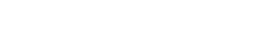14(k+12) – 9(2k+16) = 4M1 for equating det.to 4
14k-18k+168-144= 4
-4k=4-24
-4k=-20
K=5
b)  det = (5 x 9 - 6 x 7)
45 - 42
= 320

(x+)(x-2) = 24 x 1
x2 – 2x + 3x – 6 = 24
x2 + x - =24
x2 + x – 30 = 0
P = -30    6,5
S = -1
x2 + 6x – 5x – 30 = 0
x(x+6) – 5(x+6) + 0
(x – 5) (x + 6) = 0
x = 5 or -6

(b).(9+x)(x) = 36
9x + x2 = 36
x2 + 9x – 36 = 0
P = -36 (-3,12)
S = 9
x2 – 3x + 12x – 36 = 0
x(x-3)+12(x-3) =0
(x+12)(x-3) = 0
x = -12 or 3
=x = 3m
Lenth = 9+x
=12m
Width=3
P =2(12+3)
=30m

c).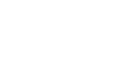36 – 2x2 = 28
2x2 = 8
x2 = 4
x = 2m

21

(a)(b) (i). GAB  =  -3 + 1 = 4 = 4
-7 - 1    -8
(ii), Equ. AB
-½ = y - 3
x + 7
2(y) – 3) = -x – 7
2y – 6 = -x – 7
2y = -x – 7 + 6
2y = -x – 1
y = - ½x – ½

c). (i) CD = - ½
GL = 2
2 =  y - 3
x - 1
2x – 2 = y – 3
y – 2x = -2 + 3
y – 2x = 1

(ii). y = 2x + 1
y intercept = 1

22

 Mark % f Mid points fx cf 40-44 4 42 168 4 45-49 7 47 329 11 50-54 8 52 416 19 55-59 6 57 342 25 60-64 7 62 434 32 65-69 3 67 201 35 70-74 5 72 360 40 75-79 0 77 0 = 40 = 2250

(b). Modal class – 50 – 54

c).(i). x = ∑fx
∑f
2250
40
= 56.25 marks

d). Median mark
m = L + (N/2 – cf)i
f
= 54.5 + (40/2 – 19)5     (B1 for of an table )
6
= 54.5 + 0.8333
= 55.3333
= 55 1/3    A1 Exact value
don’t accept 55.333/55.3

23

24

y = 2x3-9x2+px+1
(i).  dy = 62 – 18x + p
dx  6(4)2 – 18(4) + P =36
96 – 72 +P = 36
24 + P = 36
P = 36 – 24
P = 12

(ii). dy = 6x2 – 18x + 12
dx
At x = 0.5
6(0.5)2 – 18(0.5)+12
= 4.5
x = 0.5
y =2(0.5)2 – 18(0.5)2 + 12(0.5)+1
= 5
y-5 = 4.5
x–0.5
y – 5 = 4.5x – 2.25
y = 4.5x + 2.75
y = 9/2x + 23/4
6x2 – 18k + 12 = 0
x2-3x+2=0
(x-2) (x-1)=0
x = 2 or 1
When x =1 y=2(1)-9(1)+12(1)+1
= 6
(1,6) and
x = 2
y = 2(2)3-9(2)2+12(2)+1                   B1 for both
y = 5                             (2,5)

• ✔ To read offline at any time.
• ✔ To Print at your convenience
• ✔ Share Easily with Friends / Students